Question

# The uniform, normal, and exponential distributions a. are all continuous probability distributions. b. are all discrete...

The uniform, normal, and exponential distributions

 a. are all continuous probability distributions.
 b. are all discrete probability distributions.
 c. are all the same distributions.
 d. can be either continuous or discrete, depending on the data.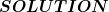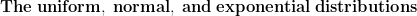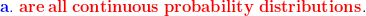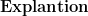1. The Uniform, Normal, and Exponential distributions are all continuous probability distributions.
2. The Binomial, Poisson, Hypergeometric distributions are all discrete probability distributions.

#### Earn Coins

Coins can be redeemed for fabulous gifts.

Similar Homework Help Questions
• ### What kind of distributions are the binomial and Poisson probability distributions? A. Discrete B. Continuous  ...

What kind of distributions are the binomial and Poisson probability distributions? A. Discrete B. Continuous   C. Both discrete and continuous D. Neither discrete or continuous

• ### Two common probability distributions are ______ and _____. A. Discrete, Continuous B. Discrete C. Continuous D....

Two common probability distributions are ______ and _____. A. Discrete, Continuous B. Discrete C. Continuous D. None of the above

• ### In 100-200 words, please explain the difference between the three continuous probability distributions: the uniform probability...

In 100-200 words, please explain the difference between the three continuous probability distributions: the uniform probability distribution, the normal probability distribution, and the exponential probability distribution.

• ### Find the 60th percentile of the following distributions: (a) Exponential with mean θ (b) Continuous uniform...

Find the 60th percentile of the following distributions: (a) Exponential with mean θ (b) Continuous uniform on [1,5] (c) f (x)= (x+1)/2 ,−1< x <1

• ### 1. Properties of the uniform, normal, and exponential distributions Aa Aa Suppose that x1 is a...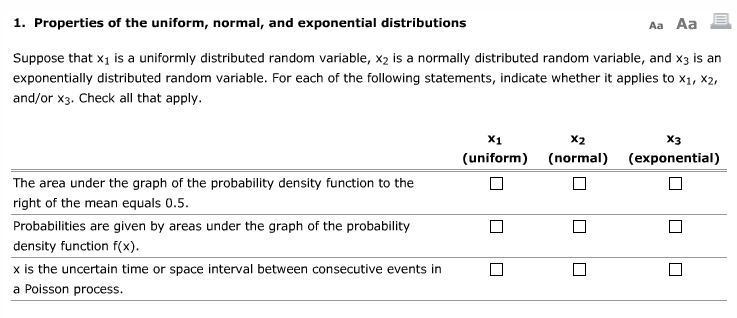1. Properties of the uniform, normal, and exponential distributions Aa Aa Suppose that x1 is a uniformly distributed random variable, x2 is a normally distributed random variable, and x3 is an exponentially distributed random variable. For each of the following statements, indicate whether it applies to x1, x2, and/or x3. Check all that apply. x2 x3 (uniform) (normal) (exponential) The area under the graph of the probability density function to the right of the mean equals 0.5. Probabilities are given...

• ### 1- Evaluate Normality and analyze all continuous distributions are not normal? 2- Analyze that th...

1- Evaluate Normality and analyze all continuous distributions are not normal? 2- Analyze that the uniform distributionis a probability distribution that has equal probabilitiesfor all possible outcomes of the random variable?

• ### Discrete Probability Distributions, Continuous Probability Distri- butions, and Sampling Distributions (100 points) 1. Does each of...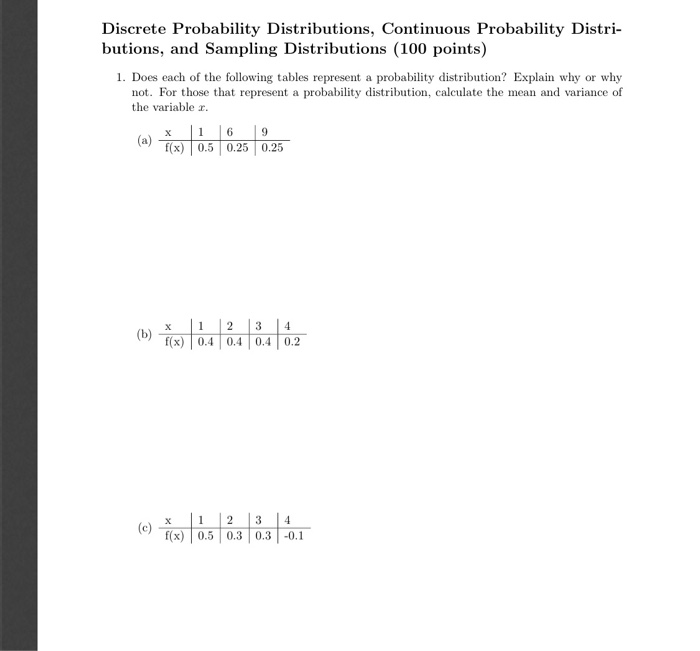Discrete Probability Distributions, Continuous Probability Distri- butions, and Sampling Distributions (100 points) 1. Does each of the following tables represent a probability distribution? Explain why or why not. For those that represent a probability distribution, calculate the mean and variance of the variable r. a f(x) 0.5 0.25 0.25 f(x) 0.4 0.4 0.4 0.2 ( X 1 2 3 4 C) f(x) 0.5 0.3 0.3 -0.1

• ### 1. A statistics professor posted 10 review problems on probability distributions: 4 problems were on discrete...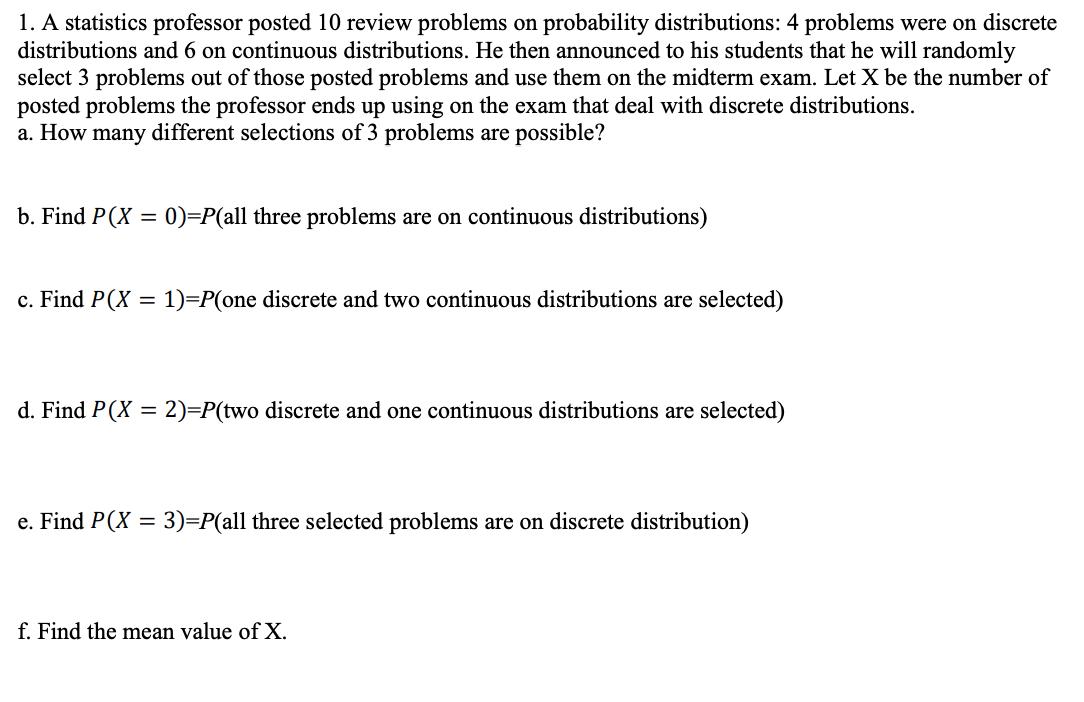1. A statistics professor posted 10 review problems on probability distributions: 4 problems were on discrete distributions and 6 on continuous distributions. He then announced to his students that he will randomly select 3 problems out of those posted problems and use them on the midterm exam. Let X be the number of posted problems the professor ends up using on the exam that deal with discrete distributions. a. How many different selections of 3 problems are possible? b. Find...

• ### Question 1 1 pts Normal distribution is Discrete o Continuous Binomial Exponential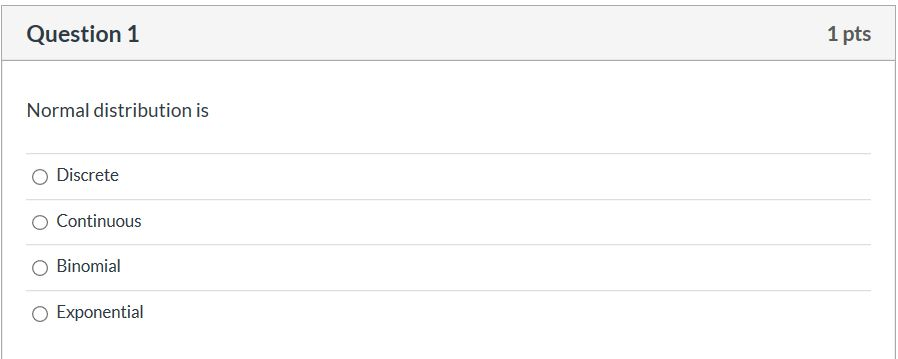Question 1 1 pts Normal distribution is Discrete o Continuous Binomial Exponential

• ### If requirements hold true, the distribution of the sample means is, Exponential, Uniform, Normal, OR Discrete?

If requirements hold true, the distribution of the sample means is, Exponential, Uniform, Normal, OR Discrete?